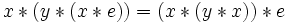# Left Bol implies flexible

This article gives the statement and possibly, proof, of an implication relation between two algebra loop properties. That is, it states that every algebra loop satisfying the first algebra loop property (i.e., left Bol loop) must also satisfy the second algebra loop property (i.e., flexible loop)
View all algebra loop property implications | View all algebra loop property non-implications
Get more facts about left Bol loop|Get more facts about flexible loop
This article gives the statement and possibly, proof, of an implication relation between two magma properties. That is, it states that every magma satisfying the first magma property (i.e., left Bol magma with neutral element) must also satisfy the second magma property (i.e., flexible magma)
View all magma property implications | View all magma property non-implications
Get more facts about left Bol magma with neutral element|Get more facts about flexible magma

## Statement

### Verbal statement

Any left Bol loop is a flexible loop.

### Slightly more general version for magmas

Any Left Bol magma with neutral element (?) (i.e., a Left Bol magma (?) with a Neutral element (?)) is a Flexible magma (?).

## Proof

We prove the statement for magmas; the same proof works for loops, since the other conditions needed to make a loop are undisturbed.

Given: A magma$(L,*)$ with neutral element$e$ satisfying the following identity for all$x,y,z \in L$:$\! x * (y * (x * z)) = (x * (y * x)) * z$.

To prove: For all$x,y \in L$, we have$(x * y) * x = x * (y * x)$.

Proof: Set$z = e$ in the identity, and we obtain:$\! x * (y * (x * e)) = (x * (y * x)) * e$

Using that$e$ is a neutral element, we obtain:$\! x * (y * x) = (x * y) * x$

as desired.